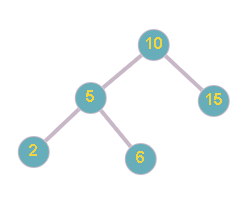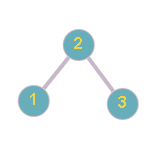New update is available. Click here to update.

# Floor in BST

Medium0/80
Avg time to solve 30 mins
Success Rate 70 %Share43 upvotes

## Problem Statement

#### For example:``````In the above example, For the given BST  and X = 7, the greatest value node of the BST  which is smaller than or equal to  7 is 6.
``````
Detailed explanation ( Input/output format, Notes, Images )##### Constraints:
``````1 <= T <= 5
1 <= N <= 5 * 10 ^ 3
1 <= nodeVal[i] <= 10 ^ 9

Time Limit: 1 sec.
``````
##### Sample Input 1:
``````2
10 5 15 2 6 -1 -1 -1 -1 -1 -1
7
2 1 3 -1 -1 -1 -1
2
``````
##### Sample Output 1:
``````6
2
``````
##### Explanation of Sample Input 1:
``````In the first test case, the BST looks like as below:
````````````The greatest value node of the BST which is smaller than or equal to  7 is 6.

In the second test case, the BST looks like as below:
````````````The greatest value node of the BST which is smaller than or equal to  2 is 2.
``````
##### Sample Input 2:
``````2
5 3 10 2 4 6 15 -1 -1 -1 -1 -1 -1 -1 -1
15
5 3 10 2 4 6 15 -1 -1 -1 -1 -1 -1 -1 -1
8
``````
##### Sample Output 2:
``````15
6
``````AutoConsole